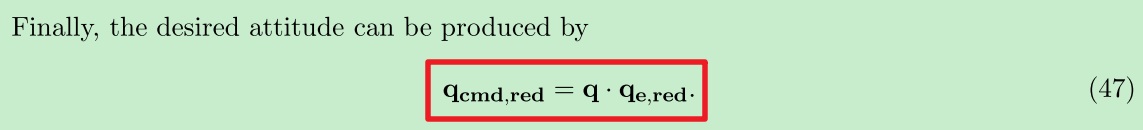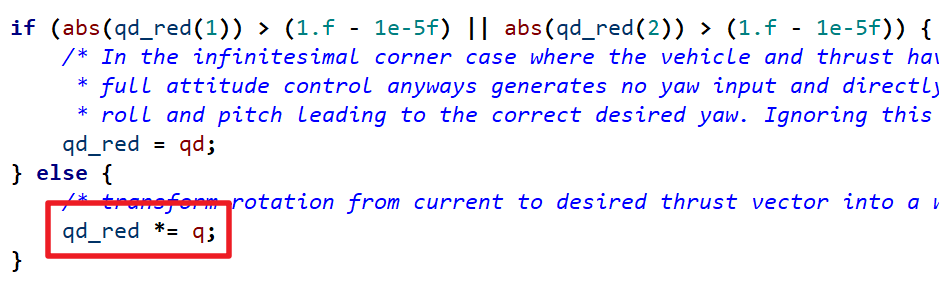# [飞控] 姿态误差 (五)-PX4 如何计算姿态误差？

1.先对齐z轴求得一个「倾斜误差」。

2.「总误差」—「倾斜误差」得到「旋转误差」。

3.对这个真实的旋转误差z做一些限制，得到一个「虚拟旋转误差」。

4.「虚拟旋转误差」加「倾斜误差」得到「虚拟总误差1.z 轴向量叉乘，得到对齐 z 轴的旋转，注意转轴还是N系

$$[Q_{half}^{cur}]^N$$

2.转轴转换到 cur 系 (这一步和APM是一模一样的哟)

$$Q_{half}^{cur}=(Q_{cur}^N)^{*} [Q_{half}^{cur}]^N (Q_{cur}^N)$$

3.计算地理系下的倾斜旋转（论文里写的其实是这一步）

$$Q_{half}^N = Q_{cur}^N Q_{half}^{cur}$$

4.计算旋转误差

$$Q_{tar}^{half}=(Q_{half}^N)^*Q_{tar}^N$$

5.使用固定系数yaw_w 限制 旋转误差

$$q_{tar}^{half}=Q_{tar}^{half}*yaw_w$$

1.在倾转分离后，PX4 采用了 「衰减」系数的形式来限制「倾斜误差

2.使用四元数乘法还原「虚拟总误差」，一定可以对其 z 轴。

3.不知道为什么新算法采用固定的「衰减」系数，之前是根据当前的 roll 和 pitch 计算变化的系数

ok，今天就讲这么多，下期我会使用 MATLAB 看看这个算法的直观效果，我是zing，一个有趣的算法工程师，我们下期见。

ps:为了方便感兴趣的朋友，给出这部分代码的注释，方便对照

MulticopterAttitudeControl::control_attitude()
{
vehicle_attitude_setpoint_poll();

_thrust_sp = -_v_att_sp.thrust_body;

//zing-note:modules\mc_att_control\mc_att_control_params.c
//zing-note:根据增益求偏航权重
Vector3f attitude_gain = _attitude_p;
// 6.5    6.5   2.8
const float roll_pitch_gain = (attitude_gain(0) + attitude_gain(1)) / 2.f;
const float yaw_w = math::constrain(attitude_gain(2) / roll_pitch_gain, 0.f, 1.f);
attitude_gain(2) = roll_pitch_gain;
//zing-note: 目标到初始
Quatf q(_v_att.q);
Quatf qd(_v_att_sp.q_d);
//zing-note: 归一化
q.normalize();
qd.normalize();
//zing-note:z轴转到机体系
Vector3f e_z = q.dcm_z();
Vector3f e_z_d = qd.dcm_z();
//zing-note:Chalf->cur
Quatf qd_red(e_z, e_z_d);
if (abs(qd_red(1)) > (1.f - 1e-5f) || abs(qd_red(2)) > (1.f - 1e-5f)) {
//zing-note:没有倾斜误差的情况
qd_red = qd
} else {
//zing-note:qd_red = Qhalf->N  =  Qcur->half * Qcur->n
qd_red *= q;
}
//zing-note:q_mix 旋转误差  Qtar->half = (Qhalf->n)^-1 * Qtar->n
Quatf q_mix = qd_red.inversed() * qd;
q_mix *= math::signNoZero(q_mix(0));
q_mix(0) = math::constrain(q_mix(0), -1.f, 1.f);
q_mix(3) = math::constrain(q_mix(3), -1.f, 1.f);
//zing-note:限制旋转误差  组合成新的旋转 qd= Qhalf->N * Qtar->half = Qtar->N
qd = qd_red * Quatf(cosf(yaw_w * acosf(q_mix(0))), 0, 0, sinf(yaw_w * asinf(q_mix(3))));
//zing-note:计算出[虚拟总误差]
Quatf qe = q.inversed() * qd;

//zing-note:后面的可以战胜不管
Vector3f eq = 2.f * math::signNoZero(qe(0)) * qe.imag();
_rates_sp = eq.emult(attitude_gain);
_rates_sp += q.inversed().dcm_z() * _v_att_sp.yaw_sp_move_rate;
/* limit rates */
for (int i = 0; i < 3; i++) {
if ((_v_control_mode.flag_control_velocity_enabled || _v_control_mode.flag_control_auto_enabled) &&
!_v_control_mode.flag_control_manual_enabled) {
_rates_sp(i) = math::constrain(_rates_sp(i), -_auto_rate_max(i), _auto_rate_max(i));

} else {
_rates_sp(i) = math::constrain(_rates_sp(i), -_mc_rate_max(i), _mc_rate_max(i));
}
}
}